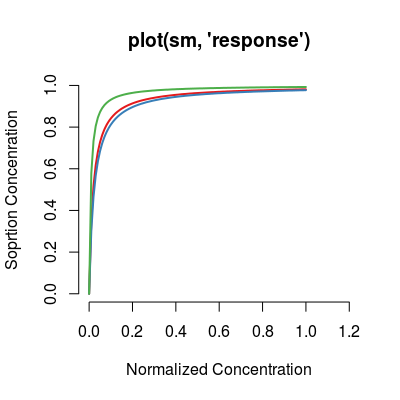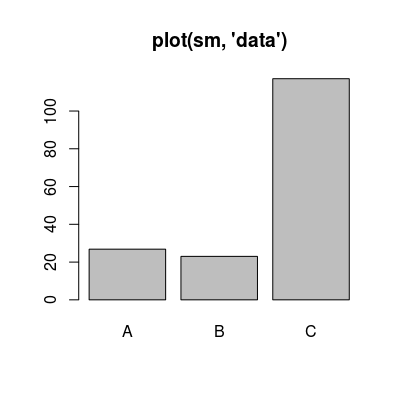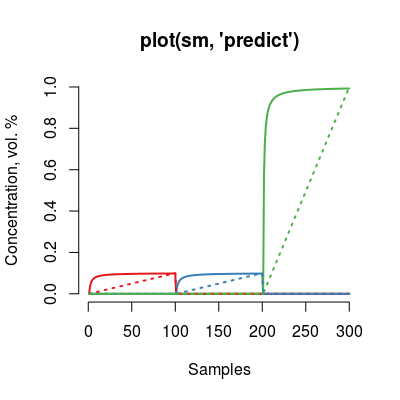# Method knum.

## Usage

```defaultSorptionModel()

SorptionModel(...)```

## Arguments

...
parameters of constructor.

## Value

List of the default parameters.

## Description

Method knum.

Method concUnitsSorption.

Class `SorptionModel` controls the the amount of gas absorbed by the sensor, that emulates the non-linearity nature intrinsic for the polymeric sensors.

Function to get default constructor parameters of class `SorptionModel`.

Constructor method of SorptionModel Class.

Wrapper function SorptionModel.

## Details

The model is based on the extended Langmuir isotherm for a multi-component gas mixture, and has two parameters per analyte, `Q` denotes the sorption capacity and `K` stands for the sorption affinity.

Slots of the class:

 `knum` `sorptionModel` List of model parameters 'K' and 'Q'.
Slots of the class:
 `knum` Sensor number that encodes a UNIMAN sorption profile (`1:17`). The default value is `1`. `gases` Gas indices. `ngases` The number of gases. `gnames` Names of gases. `concUnits` Concentration units external to the model, values given in an input concentration matrix. `concUnitsInt` Concentration units internal for the model, values used numerically to evaluate the Langmuir relation. `sorptionModel` A list that contains the Langmuir parameters. `srdata` The reference data of Lanmuir parameters from UNIMAN dataset (see `UNIMANsorption`). `alpha` (parameter of sensor non-linearity in mixtures) A scaling coefficient of non-linearity induced via the affinity parameter `K`. The default value is `2.25`.

Methods of the class:

 `predict` Predicts a model response to an input concentration matrix.

The `plot` method has three types (parameter `y`):

 `response` (default) Shows a modeled trasnformation of concentration profile per analyte. `data` Shows the reference data from UNIMAN dataset. `predict` Depicts input and ouput of the model for all analytes.

## Note

We introduce a single parameter `alpha` of the model to control the level of non-linearity simulated by the Langmuir isotherm. This parameter `alpha` defines a normalization across the 17 UNIMAN sorption profiles from dataset `UNIMANsorption`, scaling `K` values based on other two parameters `Kmin` (default value `1` and `Kmax` (default value `150`). Normalization can be disable by setting parameter `Knorm` to `FALSE`, that results in usage of the sorption `K` parameters, equal to UNIMAN ones.

## Examples

```# sorption model: default initialization
sm <- SorptionModel()

# get information about the model
show(sm)

Sorption Model (knum 1), alpha 2.25

print(sm)

Sorption Model
- knum 1
- 3 gases A, B, C

plot(sm)# model: custom parameters
# almost linear model
sm <- SorptionModel(alpha=0.01, gases=c(1, 3))
plot(sm, main="Almost linear model, non-linearity 0.01")# non-linear model
sm <- SorptionModel(alpha=0.3, gases=c(1, 3))
plot(sm, main="Non-linear model, non-linearity 0.5")# saturated model
sm <- SorptionModel(alpha=0.5, gases=c(1, 3))
plot(sm, main="Saturated model, non-linearity 1")# model with UNIMAN sorption parameters
sm <- SorptionModel(Knorm=FALSE, gases=c(1, 3))
plot(sm, main="Model with UNIMAN parameters (no normalization)")# method plot
#  - plot types 'y': response, data, predict
sm <- SorptionModel() # default model

plot(sm, "response", main="plot(sm, 'response')")# default plot type, i.e. 'plot(sm)' does the same plotting

plot(sm, "data", main="plot(sm, 'data')")plot(sm, "predict", main="plot(sm, 'predict')")```

`UNIMANsorption`